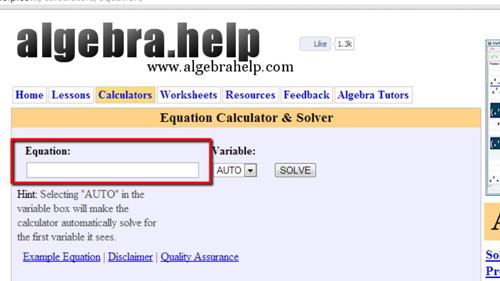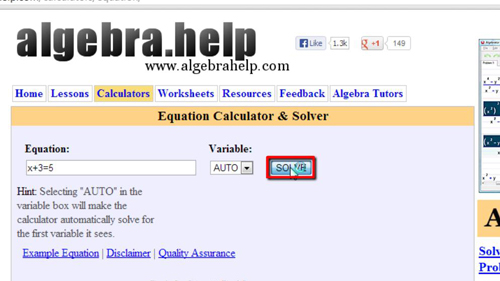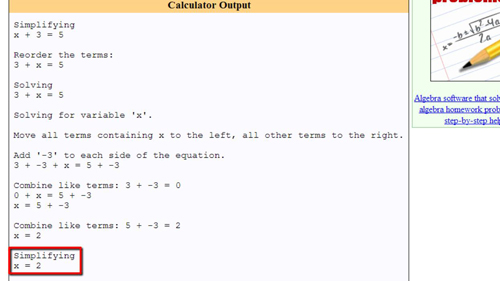## How to Use Formula Calculator

In this tutorial you are going to learn how to use a formula calculator online which can be a very easy way to work out equations with minimal effort.

Step # 1 – Navigating to the Formula Site

First you are going to need to open the website that will allow you to work out a formula. Visit “http://www.algebrahelp.com/calculators/equation”. On this site you can see an entry box at the top that will allow you to enter an equation as well as select a variable.Step # 2 – Entering the Equation

In this example we are going to enter a simple formula with a variable of “x”. We will type “x+3=5”. You can choose a variable from the drop down box if need be, but as we only have one variable here, we don’t need to do this and can leave the setting on “AUTO”. Click the “SOLVE” button when you are ready.Step # 3 – Viewing the Result

On the next page a solution will load and you can see at the bottom that the calculator has correctly worked out that “x=2”. Unlike most calculators this one shows details working which is particularly useful if you are learning how to work out these equations for yourself. That is essentially how to use a formula calculator online.